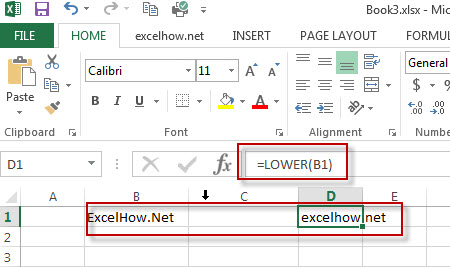# Excel Lower Function

This post will guide you how to use Excel LOWER function with syntax and examples in Microsoft excel.

### Description

The Excel LOWER function converts all characters in a text string to lowercase.

The LOWER function is a build-in function in Microsoft Excel and it is categorized as a Text Function.

The LOWER function is available in Excel 2016, Excel 2013, Excel 2010, Excel 2007, Excel 2003, Excel XP, Excel 2000, Excel 2011 for Mac.

### Syntax

The syntax of the LOWER function is as below:

= LOWER  (text)

Where the LOWER function arguments are:
text -This is a required argument. The text string that you want to convert to lowercase.

### Example

The below examples will show you how to use Excel LOWER Text function to convert all uppercase characters in a text string to lowercase.

#1 To lower case all characters in B1 cell, just using the following formula:=LOWER(B1).### More Related LOWER Function Examples

• Change Uppercase and Lowercase Text
if you want to change all text string in uppercase into lowercase, you can create a formula based on the Lower function. And if you want to convert all letters in one cell into uppercase, you can use the Upper function as a formula in excel.…